# Test: Reaction Mechanism Level - 6

## 24 Questions MCQ Test Organic Chemistry | Test: Reaction Mechanism Level - 6

Description
Attempt Test: Reaction Mechanism Level - 6 | 24 questions in 50 minutes | Mock test for Chemistry preparation | Free important questions MCQ to study Organic Chemistry for Chemistry Exam | Download free PDF with solutions
*Multiple options can be correct
QUESTION: 1

### Which of the following statement(s) is/are true about the reaction given below: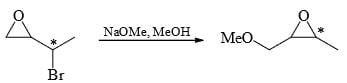(1) it involves a carbocation intermediate (2) rearrangement is due to SN1 reaction mechanism. (3) it proceeds via a concerted SN2 pathway (4) it involves neighbouring group participation.

Solution: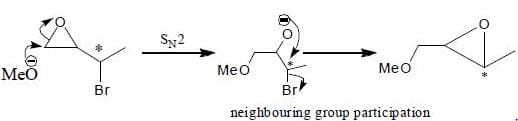From above mechanism:
If proceecs via a concerted SN2 pathway.
It involves neighbouring group participation.

*Multiple options can be correct
QUESTION: 2

### The reaction (s) which give (s) phenol is/are:

Solution:
*Multiple options can be correct
QUESTION: 3

### Compound(s) that on hydrogenation product(s) optically inactive compound(s) is(are):

Solution:
*Multiple options can be correct
QUESTION: 4

When 20 g of a compound (A) (M.F. = C4H10O4) reacts with excess of CH3MgBr, 14.6 L of CH4 is obtained at STP. What is structural formula of (A)?

Solution:
*Multiple options can be correct
QUESTION: 5

In the following reaction, the product(s) formed is (are)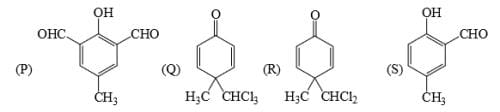Solution:
*Multiple options can be correct
QUESTION: 6

The major product(s) of the following reaction is (are):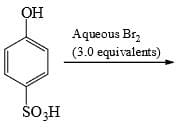Solution: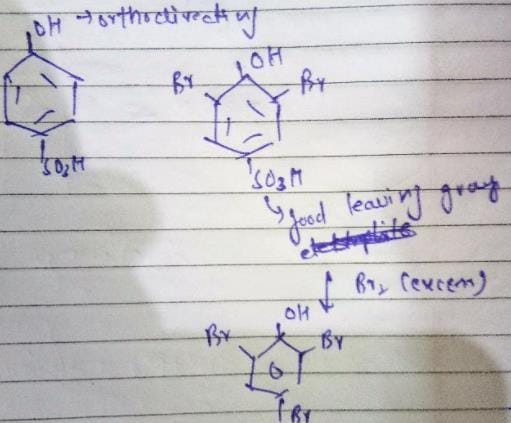*Multiple options can be correct
QUESTION: 7

Which of the following ethers can be synthesized directly by Williamson’s synthesis:

Solution:
*Multiple options can be correct
QUESTION: 8

Among P, Q, R and S the aromatic compound(s) is/are:

Solution:
*Multiple options can be correct
QUESTION: 9

The correct statement(s) about the following reaction sequence is/are: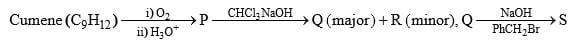Solution:
*Multiple options can be correct
QUESTION: 10

For the following compounds, the correct statement(s) with respect to nucleophile substitution reaction is(are):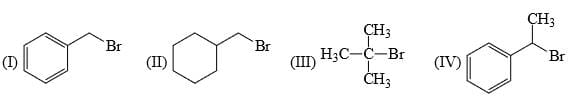Solution:
*Multiple options can be correct
QUESTION: 11

Compounds P and R upon ozonolysis produce Q and S, respectively. The molecular formula of Q and S is C8H8O. Q undergoes Cannizzaro reaction but not Haloform reaction, whereas S undergoes Haloform reaction but no Cannizzaro reaction.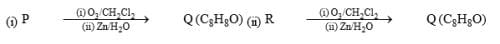The option(s) with suitable combination of P and R, respectively, is(are)

Solution:

The options (A) and (B) are with suitable combination of P and R, respectively.
When no alpha H atom is present, aldehyde will undergo Cannizzaro reaction. When alpha H atom is present, aldehyde will not undergo Cannizzaro reaction. If it is a methyl ketone, it will undergo haloform reaction.
If it is not a methyl ketone, it will not undergo haloform reaction.
In options (C) and (D), the product on ozonolysis is not having molecular formula C8​H8​O .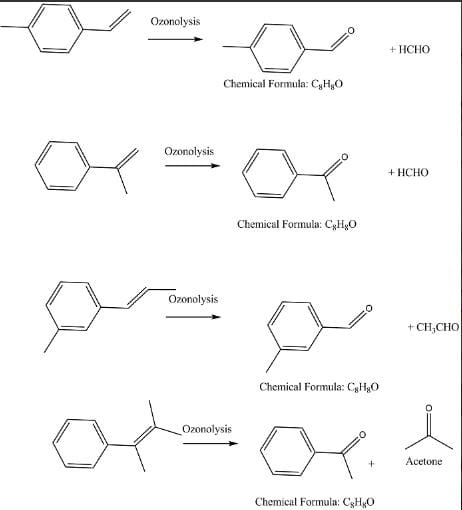*Multiple options can be correct
QUESTION: 12

The reactivity of compound Z with different halogens under appropriate conditions is given below: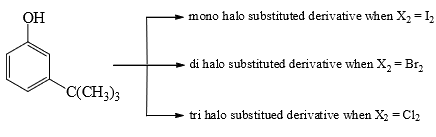The observed pattern of electrophilic substitution can be explained by:

Solution:
*Multiple options can be correct
QUESTION: 13

In the reaction shown below, the major product(s) formed is/are: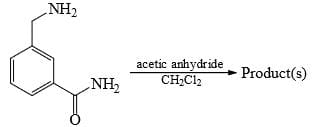Solution:
QUESTION: 14

Choose the correct one which will react faster in the SN2 nucleophilic substitution reaction?

Solution:

Ans: b

Explanation: The carbocation character in the transition state causes stabilization of the resonance and hence CH2 = CH – CH2 – Br (2-bromobutane) is the one which will react faster compared to the others.

*Multiple options can be correct
QUESTION: 15

Which of the following compound gives even number of Hoffmann’s exhaustive methylation and elimination?

Solution:
QUESTION: 16

Alcohol on refluxing with Cr2O7 gives ____________

Solution:

Ans: d

Explanation: Alcohol (R-OH), when it is refluxed with Cr2O7, it forms carboxylic acid (R-COOH).

*Multiple options can be correct
QUESTION: 17

Which of the following compounds will react with R-SH in aqueous solutions between pH 6.5 and 8.5: [TIFR 2012]

Solution:
*Multiple options can be correct
QUESTION: 18

After completion of the reactions (I and II), the organic compound(s) in the reaction mixture is(are):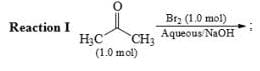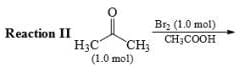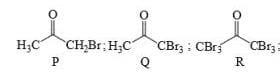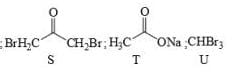Solution: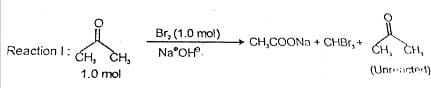( In basic medium complete haloform takes place since the rate of reaction increase with each α- halogenation )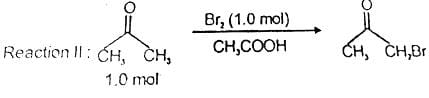(In acidic medium monohalogenation takes place with 1- mol of halogen )

*Multiple options can be correct
QUESTION: 19

Positive Tollen’s test is observed for:

Solution:
*Multiple options can be correct
QUESTION: 20

Identify the binary mixture(s) that can be separated into individual compounds, by differential extraction, as shown in the given scheme.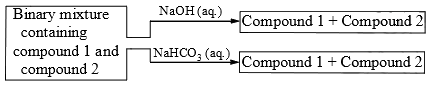Solution:
*Multiple options can be correct
QUESTION: 21

With reference to the scheme given, which of the given statement(s) about T, U, V and W is (are) correct: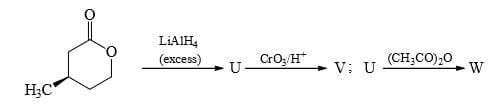Solution:
*Multiple options can be correct
QUESTION: 22

Which of the following compounds will react with ethanolic KCN:

Solution:
*Multiple options can be correct
QUESTION: 23

A new C—C bond formation is possible in:

Solution:
*Multiple options can be correct
QUESTION: 24

In the reaction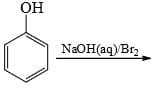the intermediate(s) is/are:

Solution:Use Code STAYHOME200 and get INR 200 additional OFF Use Coupon Code